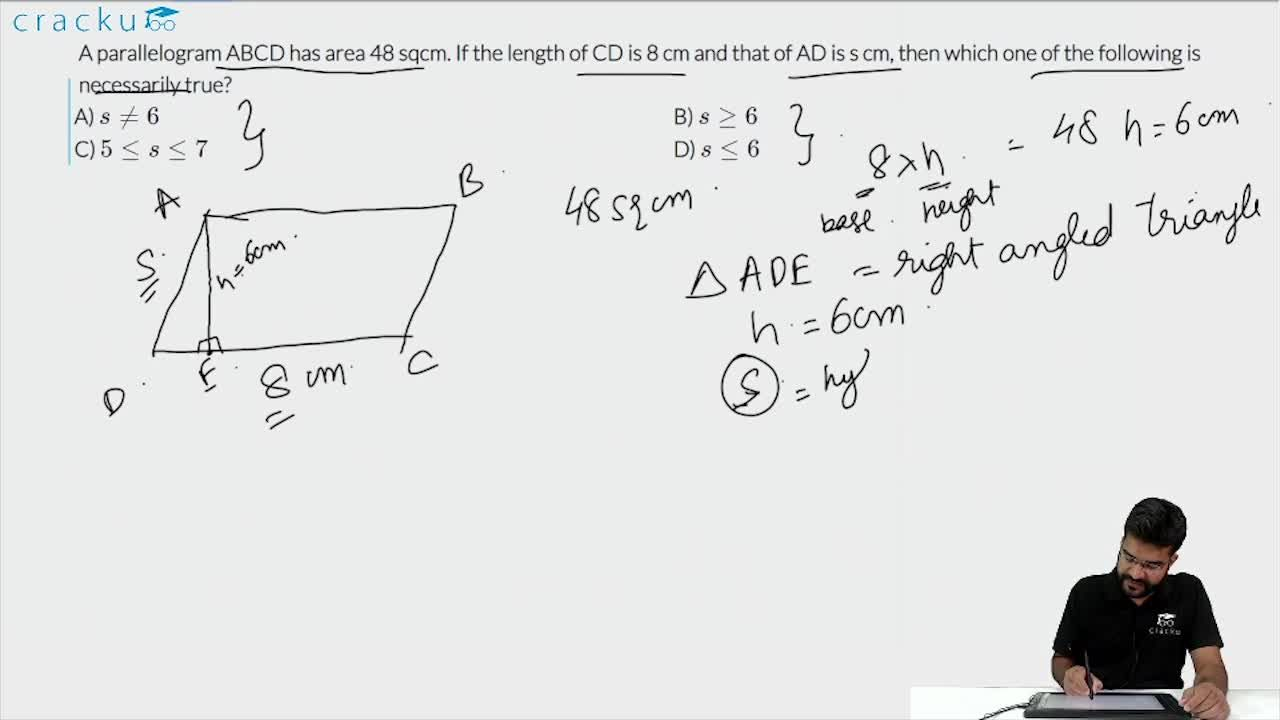Question 8

# A parallelogram ABCD has area 48 sqcm. If the length of CD is 8 cm and that of AD is s cm, then which one of the following is necessarily true?

Solution

We can see that area of parallelogram ABCD = 2*Area of triangle ACD

48 = 2*Area of triangle ACD

Area of triangle ACD = 24

$$(1/2)*CD*DA*sinADC=24$$

$$AD*sinADC=6$$

We know that $$sin\theta$$ $$\leq$$ 1, Hence, we can say that AD $$\geq$$ 6

$$\Rightarrow$$ s $$\geq$$ 6

### View Video Solution